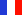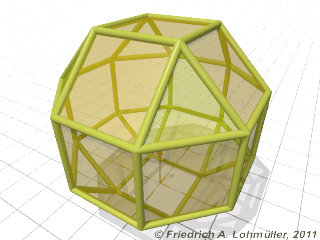###### Descriptions and Examples for the POV-Ray Raytracerby Friedrich A. Lohmüller Elementary Geometry for Raytracing
ItalianoFrançaisDeutschHome
- POV-Ray Tutorial

- Geometrical Basics
for Raytracing

Right-angled Triangle
Pythagorean Theorem
Trigonometry Basics
Law of cosines
Equilateral Triangle
Regular Polygon
Polyhedron
Tetrahedron
Octahedron
Cube & Cuboid
Dodecahedron
Icosahedron
Cuboctahedron
Truncated Octahedron
Rhombicuboctahedron
Truncated Icosahedron
Circles
Tangent circles
Internal Tangents
External Tangents

- Geometric 3D Animations## Rhombicuboctahedron(small Rhombicuboctahedron)Some useful geometrical facts.Folding of a Rhombicuboctahedron
In the following we write for the square root of a number
the expression "sqrt(
ZAHL)"
conforming to the syntax used in POV-Ray.

 Dimensions Length of an edge: a. The radius of circumsphere: R = a / 2 * sqrt( 5 + 2*sqrt(2)); The radius of edgesphere (tangent to edges): Re = a / 2 * sqrt( 4 + 2*sqrt(2) ); Coordinates of the corners: all permutations of ( +/-1, +/-1, +/-(1+sqrt(2)) ); The angle between square and square: 135° SS_Angle = degrees(acos(-1/sqrt(2))); The angle between square and triangle: ~144,74° ST_Angle = degrees(acos(-sqrt(2/3))); The angle between edges: 135° Edge_Angle = degrees(acos(-1/sqrt(2)));Rhombicuboctahedron
top

 © Friedrich A. Lohmüller, 2011 http://www.f-lohmueller.de